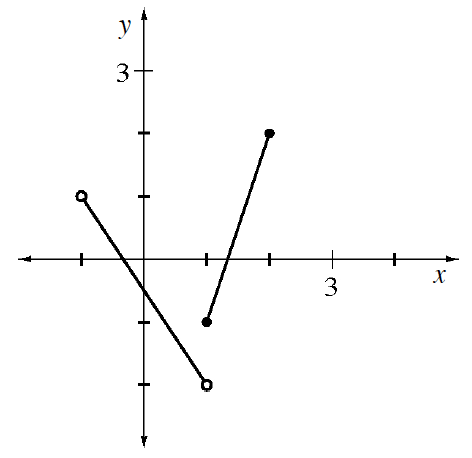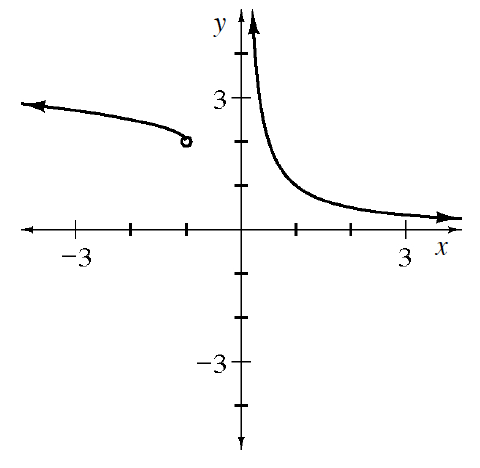### Home > APCALC > Chapter 1 > Lesson 1.3.3 > Problem1-126

1-126.

Identify the domain and range of each of the functions below. Then write a possible piecewise-defined function for each graph.

1.Domain is the set of values that x can be, while range is the set of values that $y$ can be.

Regarding the piecewise function:
Each piece has its own domain; be careful about open or closed intervals. Notice that both pieces are linear.

1.Notice that this graph has both a vertical and a horizontal asymptote, which may affect the domainand range respectively.

Regarding the piecewise function:

$\text{Notice that the left piece is a transformation of }y=\sqrt{x}$

$\text{while the right piece appears to be } y=\frac{1}{x}.$

Note that there is a gap between the two functions. So the boundary point on the left will be different from the boundary point on the right